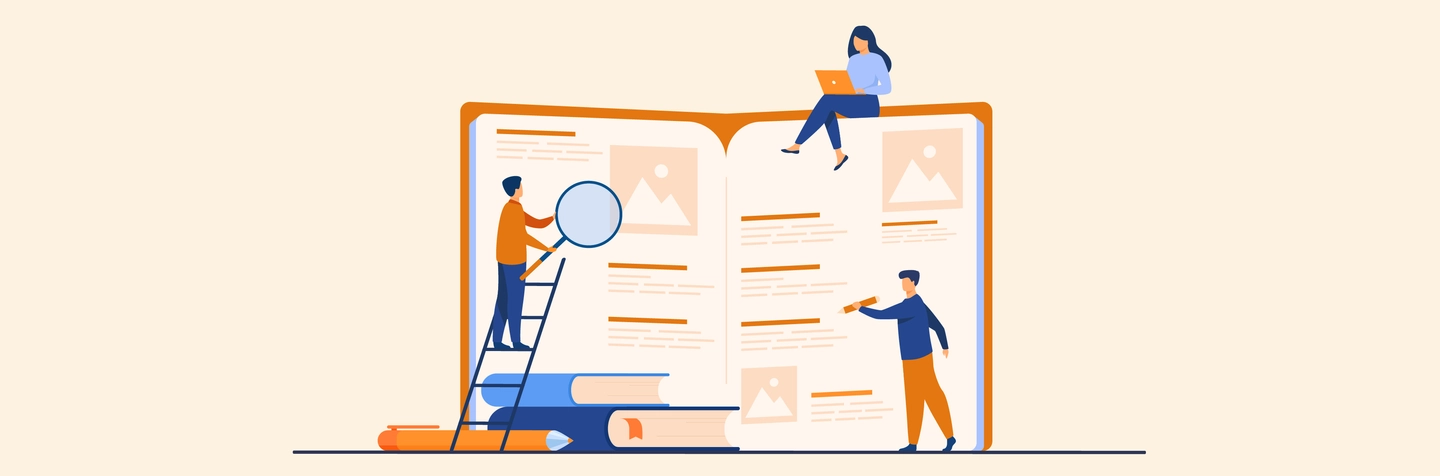Exams Know-how

# GMAT Quants Syllabus 2022: Guide to Updated GMAT Maths Syllabus7 views

MBA aspirants from all over the world take the GMAT exam to gain admission to their ideal business school. The Graduate Management Admissions Test (GMAT) has a total of 91 questions, both objective and subjective, with a maximum score of 800. The test takes three and a half hours.

The four competencies that make up the GMAT are verbal, quantitative, reasoning, and writing, but for the purposes of this blog, we are going to discuss the GMAT Quantitative Aptitude Syllabus. Keep reading to find out about the GMAT Quantitative problems that are designed to test your ability to interpret data and derive conclusions from them.

## Brief Overview Of GMAT Quantitative Syllabus

Foundational mathematical skills are assessed in the GMAT maths syllabus. Math principles taught in school are tested, along with the candidate's ability to reason quantitatively in GMAT maths syllabus. In this section you'll be assessed on how to work with numbers, how to reason quantitatively, and how to understand charts and graphs.

The quantitative section of the examination is designed to evaluate a candidate's capacity for analysis and reasoning in the context of given data. In order to be successful in the quantitative section of the exam, one needs to have a solid understanding of the mathematics covered in secondary school.

## What To Expect In The GMAT Quants Section?

Data sufficiency and problem solving are the two sections of the quantitative section. You will have 62 minutes to answer each question. There will be 18 questions each in the data sufficiency and problem solving sections of the exam. A multiple-choice format will be used for the questions.

### Problem Solving

The Problem Solving portion of the GMAT quantitative exam accounts for fifty per cent of the total problems in that section. It evaluates candidates based on their ability to apply logical and analytic thinking in the context of problem-solving with numbers.

### Data Sufficiency

Candidates are evaluated based on how well they can analyse a quantitative problem, establish which data points are significant, and pinpoint the point at which there is enough amount of information to address the problem.

## Top Factors To Keep In Mind When Solving The GMAT Quantitative Section

1. The capacity for quantitative reasoning, the solving of quantitative problems, and the interpretation of graphical data
2. Solving issues requiring knowledge of basic arithmetic, geometry and rudimentary algebra.
3. Quantitative problem-solving methodology involving an analysis of the amount of information required
4. Analysing a quantitative problem
5. Identifying which pieces of information are most pertinent
6. To have the ability to determine when there is a sufficient amount of information to solve a problem.
7. Powers and roots and fractions and statistics are all included in fundamental arithmetic.
8. Under algebra in the GMAT maths syllabus, concepts such as variables and functions are covered.
9. Triangles, circles, quadrilaterals, solids, and cylinders are all examples of geometric objects, and their properties come under geometry.
10. Using geometric concepts and a combination of arithmetic and algebra to answer word problems

## Consolidated List of GMAT Quant Section Topics

Here are all the topics that will be covered in the GMAT quants syllabus:

 Category Subcategory Arithmetic Probability Ratio and proportion Simple and Compounded Interest Speed, time, distance Percentage Average Fractions Decimals Number Properties Multiples and Factors Algebra Permutation and combination Algebraic expressions and equations Arithmetic and geometric progressions Statistics Exponents Functions Geometry Coordinate geometry Circles Quadrilaterals Triangle Lines and angles

GMAT quants syllabus has a lot of material. It includes word problems, quadratic equations, data sufficiency, and problem-solving questions. The GMAT quants syllabus is a great place to start your GMAT prep strategy. Preparing for the GMAT in two months requires dedication and consistency. For more information on GMAT Quantitative Syllabus or about studying abroad, reach out to our Yocket counsellors today!

Ques. Is GMAT Quant difficult?

Ans. No, the quant section of the GMAT is not difficult. Quant on the GMAT is more logical and requires fewer calculations.

Ques. What are the subjects in GMAT?

Ans. The four components of the GMAT exam are Analytical Writing, integrated Reasoning, Quantitative Reasoning and  Verbal Reasoning.

Ques. Which section of GMAT is hardest?

Ans. Most test takers find the data sufficiency questions under Quants section the hardest to attempt.

Ques. What level math is on GMAT?

Ans. Secondary school math is tested on the GMAT.

Ques. Is calculus included in GMAT?

Ans. No, calculus is not tested on the GMAT.

Articles you might like
View All